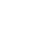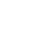LV. 41
GP 3k(只要500Kbps約每秒50kb的上傳，和低CPU使用率，就能達到不錯的畫質)

↑特別針對LoL類型的遊戲下去做優化

*(因1600kbps + 聲音的最大值400kbps = 2000kbps)

==============悲劇低階電腦專用====適用於1500Kbps++==========

Low#1
8x8dct=1 aq-mode=2 bframes=1 chroma-qp-offset=1 colormatrix=smpte170m deblock=0:0 direct=auto ipratio=1.41 keyint=240 level=3.1 me=hex merange=16 min-keyint=auto mixed-refs=1 no-mbtree=0 partitions=i4x4,p8x8,b8x8 profile=main psy-rd=0.5:0.0 qcomp=0.6 qpmax=51 qpmin=10 qpstep=4 ratetol=10 rc-lookahead=30 ref=1 scenecut=40 subme=1 threads=0 trellis=2 weightb=1 weightp=2

Low2

=================中低～中～高階電腦專用===適用於1000Kbps+============

a為需要最不損cpu資源的

a
8x8dct=1 aq-mode=2 bframes=1 chroma-qp-offset=1 colormatrix=smpte170m deblock=0:0 direct=auto ipratio=1.41 keyint=240 level=3.1 me=hex merange=16 min-keyint=auto mixed-refs=1 no-mbtree=0 partitions=i4x4,p8x8,b8x8 profile=main psy-rd=0.5:0.0 qcomp=0.6 qpmax=51 qpmin=10 qpstep=4 ratetol=10 rc-lookahead=30 ref=1 scenecut=40 subme=5 threads=0 trellis=2 weightb=1 weightp=2

b
8x8dct=1 aq-mode=2 bframes=1 chroma-qp-offset=1 colormatrix=smpte170m deblock=0:0 direct=auto ipratio=1.41 keyint=240 level=3.1 me=hex merange=16 min-keyint=auto mixed-refs=1 no-mbtree=0 partitions=i4x4,p8x8,b8x8 profile=main psy-rd=0.5:0.0 qcomp=0.6 qpmax=51 qpmin=10 qpstep=4 ratetol=10 rc-lookahead=30 ref=1 scenecut=40 subme=6 threads=0 trellis=2 weightb=1 weightp=2

c
8x8dct=1 aq-mode=2 bframes=1 chroma-qp-offset=2 colormatrix=smpte170m deblock=0:0 direct=auto ipratio=1.41 keyint=240 level=3.1 me=hex merange=16 min-keyint=auto mixed-refs=1 no-mbtree=0 partitions=i4x4,p8x8,b8x8 profile=main psy-rd=0.5:0.0 qcomp=0.6 qpmax=51 qpmin=10 qpstep=4 ratetol=10 rc-lookahead=30 ref=1 scenecut=40 subme=7 threads=0 trellis=2 weightb=1 weightp=2

d
8x8dct=1 aq-mode=2 b-adapt=2 bframes=1 chroma-qp-offset=2 colormatrix=smpte170m deblock=0:0 direct=auto ipratio=1.41 keyint=240 level=3.1 me=umh merange=16 min-keyint=auto mixed-refs=1 no-mbtree=0 partitions=all profile=high psy-rd=0.5:0.0 qcomp=0.6 qpmax=51 qpmin=10 qpstep=4 ratetol=10 rc-lookahead=30 ref=1 scenecut=40 subme=8 threads=0 trellis=2 weightb=1 weightp=2

e
8x8dct=1 aq-mode=2 b-adapt=2 bframes=1 chroma-qp-offset=2 colormatrix=smpte170m deblock=0:0 direct=auto ipratio=1.41 keyint=240 level=3.1 me=umh merange=16 min-keyint=auto mixed-refs=1 no-mbtree=0 partitions=all profile=high psy-rd=0.5:0.0 qcomp=0.6 qpmax=51 qpmin=10 qpstep=4 ratetol=10 rc-lookahead=30 ref=1 scenecut=40 subme=10 threads=0 trellis=2 weightb=1 weightp=2

===========

=================

face基於日前微軟官方表示 Internet Explorer 不再支援新的網路標準，可能無法使用新的應用程式來呈現網站內容，在瀏覽器支援度及網站安全性的雙重考量下，為了讓巴友們有更好的使用體驗，巴哈姆特即將於 2019年9月2日 停止支援 Internet Explorer 瀏覽器的頁面呈現和功能。